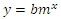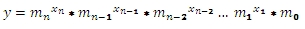# SQL Server GROWTH function

GROWTHMX

Updated: 15 November 2011

Use GROWTHMX to calculate values along an exponential trend. GROWTHMX is designed specifically for multiple x-values. If there is only one x-value for each y-value, consider using the aggregate function GROWTH.
GROWTHMX calculates the Ordinary Least Squares (OLS) solution for the given x- and y-values. The OLS solution calculates a regression analysis where an exponential curve is fitted. The known y-values are treated as dependent variables and the known x-values are treated as independent variables. For more information on the calculation of these values, see Ordinary Least Squares.
GROWTHMX computes a y-value with new x-values supplied as input to the function, using the coefficients calculated in the OLS solution.
In the case where we have one column of x-values and a column of y-values, the OLS solution is:For purposes of multi-linear regression, where there are multiple columns of x-values (and still a single column of y-values), the formula for the solution is described by the following equation:Where n is the number of x-columns and m0 is b.
The function expects the input to be in row-column, or spreadsheet, format, rather than in third-normal form. Thus, the input into the function requires the specification of the column names for the x-values and the y-values, and the specification of a 1-based index identifying the column reference for the y-values, and the specification of new x–values in the same column order as the x-values. Unlike a spreadsheet, however, there is no requirement that the columns containing the x-values be contiguous. The column specifications and the table or view that contains the data are passed into the function as strings.
Syntax
SELECT [wctStatistics].[wct].[GROWTHMX] (
<@TableName, nvarchar(4000),>
,<@ColumnNames, nvarchar(4000),>
,<@GroupedColumnName, nvarchar(4000),>
,<@GroupedColumnValue, sql_variant,>
,<@y_ColumnNumber, int,>
,<@new_x, nvarchar(4000),>
,<@Lconst, bit,>)
Arguments
@TableName
the name, as text, of the table or view that contains the values in the array to be used in the GROWTHMX calculation.
@ColumnNames
the name, as text, of the columns in the table or view specified by @TableName that contains the array values to be used in the GROWTHMX calculation. Data returned from the @ColumnNames must be of the type float or of a type that implicitly converts to float.
@GroupedColumnName
the name, as text, of the column in the table or view specified by @TableName which will be used for grouping the results.
@GroupedColumnValue
the column value to do the grouping on.
@y_ColumnNumber
the index into the array identifying the column containing the y-values. The index value must be between 1 and n, where n is the number of columns specified in @ColumnNames. @y_ColumnNumber must be of the type int or of a type that implicitly converts to int.
@new_x
The new x-values for which you want GROWTHMX to return a corresponding y-value. @new_x values must evaluate to a type of float or of a type that implicitly converts to float.
@LConst
A logical value specifying whether to force the y-intercept value (m0) equal to zero. @LConst must be of the type bit or of a type that implicitly converts to bit.
Return Types
float
Remarks
·         If @Lconst is NULL than @Lconst is set to 'True'.
·         If @Lconst is true than the number of rows must be greater than the number of columns.
·         If @Lconst is false than the number of rows must be greater than or equal to the number of columns.
·         For more complicated queries, you can try the GROWTHMX_q function.
Examples
We create a table and put some data in it.
CREATE TABLE #xy (
y     float,
x1    float,
x2    float,
x3    float
)

INSERT INTO #xy VALUES (64.744,-2,-8,0)
INSERT INTO #xy VALUES (115.589,8,3,-2)
INSERT INTO #xy VALUES (35.349,1,4,-7)
INSERT INTO #xy VALUES (77.215,-3,-1,1)
INSERT INTO #xy VALUES (28.725,-1,-3,10)
INSERT INTO #xy VALUES (54.679,5,-4,-7)
INSERT INTO #xy VALUES (27.382,-7,5,8)
INSERT INTO #xy VALUES (49.833,-8,-5,2)

This is what the data look like.
y                     x1                     x2                     x3
---------------------- ---------------------- ---------------------- ----------------------
64.744                     -2                     -8                      0
115.589                      8                      3                     -2
35.349                      1                      4                     -7
77.215                     -3                     -1                      1
28.725                     -1                     -3                     10
54.679                      5                     -4                     -7
27.382                     -7                      5                      8
49.833                     -8                     -5                      2
We have 3 columns of x-data and one column of y-data, which is in the first column of the table #xy. To invoke the table-valued function GROWTHMX, we enter the following SQL.
SELECT wct.GROWTHMX('#xy','y,x1,x2,x3','',NULL,1,'-7,9,-1','True') as GROWTH
Note that the table name and the column names are both bound by single-quotes, so that they are passed into the function as a string. Essentially the function is dynamically creating a SQL statement to SELECT the column names from the #xy table. This means that we can actually simplify the second parameter, since we are selecting all the columns in this particular table, by entering the following:
SELECT wct.GROWTHMX('#xy','*','',NULL,1,'-7,9,-1','True') as GROWTH
The third parameter is entered as blank (2 single quotes), since there is no column on which we want to group the results for input into the calculation and the fourth parameter is NULL, since there is no group column name. The fifth parameter, the y-column value, specifies that the 1st column in the resultant table, which becomes the input into the calculation, contains the y-values. The sixth parameter is the new x-values, which is entered as a comma-separated string. The last parameter specified that we want to calculate the y-intercept.
The following result is produced.
GROWTH
----------------------
31.5136692835841
By looking at the LOGEST function, we can see how GROWTHMX came up with this value. If we run the following SQL, we can mimic the TREND calculation:
SELECT *
,CASE
WHEN idx = 0 THEN 1
WHEN idx = 1 THEN -7
WHEN idx = 2 THEN 9
WHEN idx = 3 THEN -1
END as new_x
FROM wct.LOGEST('#xy','*','',NULL,1,'True')
WHERE stat_name = 'm'
This returns the following result.
stat_name          idx               stat_val       new_x
---------- ----------- ---------------------- -----------
m                    0       51.5115125618439           1
m                    1       1.03997667832838          -7
m                    2      0.974365465751811           9
m                    3      0.983415556512974          -1
With a slight modification to the SQL and using the XLeratorDB PRODUCT function, we can now reproduce the same result as GROWTHMX.
SELECT wctMath.wct.PRODUCT(POWER(stat_val, new_x)) as GROWTH
FROM (
SELECT *
,CASE
WHEN idx = 0 THEN 1
WHEN idx = 1 THEN -7
WHEN idx = 2 THEN 9
WHEN idx = 3 THEN -1
END as new_x
FROM wct.LOGEST('#xy','*','',NULL,1,'True')
WHERE stat_name = 'm'
) n
This produces the following result.
GROWTH
----------------------
31.5136692835842
Which is the result returned by GROWTHMX
In this example, we will add a new table, containing multiple new x-values, demonstrating how to calculate multiple y-values from multiple new x-values in a single SELECT.
CREATE TABLE #new_x (
r     float,
x0    float,
x1    float,
x2    float
)

INSERT INTO #new_x VALUES (1,1,1,1)
INSERT INTO #new_x VALUES (2,2,2,-2)
INSERT INTO #new_x VALUES (3,3,-3,-3)
INSERT INTO #new_x VALUES (4,-4,-4,-4)
INSERT INTO #new_x VALUES (5,-5,5,-5)
We can then run the following statement and return a new y-value for each set of new x-values.
SELECT #new_x.r
,wct.GROWTHMX('#xy','*','',NULL,1,cast(x0 as varchar)+','+cast(x1 as Varchar)+','+cast(x2 as varchar),'TRUE') as y
FROM #new_x
This produces the following result.
r                      y
---------------------- ----------------------
1       51.3318432932475
2       54.6916619602516
3       65.8564372652546
4       52.2365003841889
5       40.4306148196581
We also could have entered the following statement, using a SELECT statement to get the new x-values.
SELECT #new_x.r
,wct.GROWTHMX('#xy','*','',NULL,1,'SELECT x0, x1,x2 from #new_x where r = ' + cast(#new_x.r as varchar),'TRUE') as y
FROM #new_x
Finally, if we wanted to calculate the coefficients without a y-intercept, and supplying some different new x-values, we would modify function call to make the last parameter FALSE, as seen in the following example.
SELECT dbo.GROWTHMX('#xy','*','',NULL,1,'10,10,7','False') as GROWTH
This produces the following result.
GROWTH
----------------------
0.0780313868197243

### SupportCopyright 2008-2023 Westclintech LLC         Privacy Policy        Terms of Service